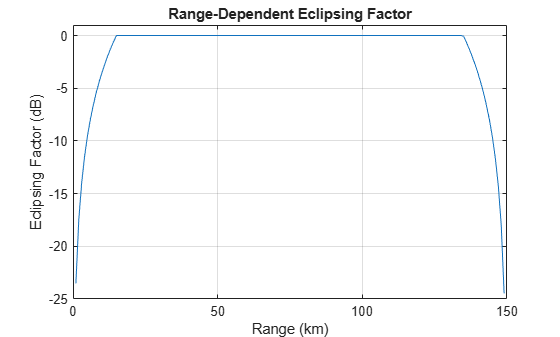# eclipsingfactor

Range-dependent eclipsing factor

## Syntax

``FECL = eclipsingfactor(R,DU,PRF)``

## Description

example

````FECL = eclipsingfactor(R,DU,PRF)` computes the range-dependent eclipsing factor `FECL` in decibels, given unambiguous range `R` duty cycle for a simple rectangular pulse or vector of samples from an arbitrary waveform `DU` and pulse repetition frequency `PRF`. ```

## Examples

collapse all

Calculate the range-dependent eclipsing factor at `1` km intervals between zero and the unambiguous range, `R`, assuming an unmodulated rectangular pulse with a duty cycle of `0.1` and the pulse repetition frequency of `1000` Hz.

```DU = 0.1; PRF = 1e3; R = 0:1000:time2range(1/PRF); FECL = eclipsingfactor(R,DU,PRF);```

Plot the range-dependent eclipsing factor.

```plot(R*1e-3,FECL) xlabel('Range (km)'); ylabel('Eclipsing Factor (dB)'); ylim([-25 1]); grid on; title('Range-Dependent Eclipsing Factor');```## Input Arguments

collapse all

Range at which to compute the eclipsing factor, specified as a positive scalar or as a length-J vector in meters.

Duty cycle, specified as a nonnegative scalar in the range `[0,1]` or length-M vector with each element in the range `[0,1]`.

• If you specify `DU` as a scalar, the eclipsing factor is computed for an unmodulated rectangular pulse with the specified duty cycle.

• If you specify `DU` as a length-M vector, the eclipsing factor is computed for a waveform, using time domain samples taken over a one-pulse interval.

Pulse repetition frequency, specified as a positive scalar or as a length-K vector in Hz.

## Output Arguments

collapse all

Eclipsing factor, returned as a J-by-K matrix in decibels with rows corresponding to the ranges in `R` and columns corresponding to the values in `PRF`.

## Extended Capabilities

### C/C++ Code GenerationGenerate C and C++ code using MATLAB® Coder™.